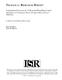## Computational Assessment of Suboptimal Bang-Bang Control Strategies for Performance-Based Design of Base Isolated Structures##### Files
TR_2005-89.pdf(555.78 KB)
This report explores the symbolic solution of the Lyapunov matrix equation as it applies to modified bang-bang control of base isolated structures. We present the Modified Bang-Bang Control strategy for active control of structures. Based on energy concepts, we formulate a rational choice of the ${ f Q}$" matrix that partitions the amount of potential energy in a base isolated system into two parts: (1) potential energy directed to the main structural system, and (2) potential energy directed to the isolation devices. This symbolic analysis of a 2-DOF system leads to investigating a choice of the ${ f Q}$ matrix that minimizes the entire potential and/or kinetic energy of a emph{n}-DOF structure during an earthquake ground event. Using symbolic analyis procedures, We show that when the entire potential and/or kinetic energy of a emph{n}-DOF structure with uniform mass is minimized, solutions to the Lyapunov matrix equation assume a greatly simplified form. Moreover, this solution to the modified bang-bang control problem is easily calculated without needing to solve the Lyapunov matrix equation. Modified bang-bang control can be easily incorporated into the second-order differential equation of motion for the structure giving intuitive insight as to the effect of active control on the response of the structure. We show that this control strategy is insensititive to localized, nonlinear stiffness changes in the base isolators and therefore is well-suited for this problem area.